# 恶意程序清理软件A-Squared

Windows
2009-10-27

EMSISoftware a-squared Anti-Malware(a2) 是一款来自奥地利，可以清理隐藏于计算机中的恶意程序的软件。它的界面简单清楚，操作容易，而且还可以随时通过在线更新最新的恶意软件资料，不怕被新出的恶意软件感染。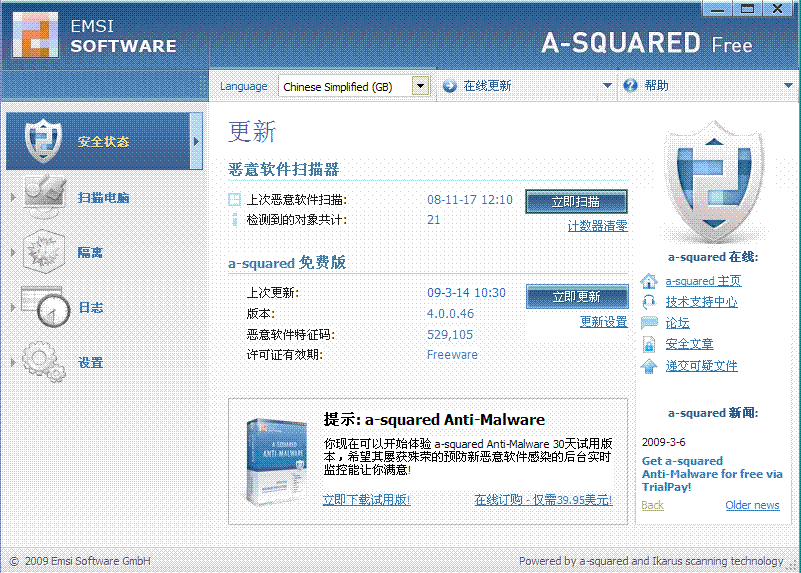### 评论(0)#### 暂无评论#### 暂无资讯#### 暂无问答

Chi-squared tests

1: Observed And Expected Frequencies In this mission, we'll be learning about the chi-squared test for categorical data. This test enables us to determine the statistical signif...

2016/09/22 18:13
3
0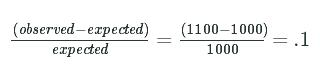Multi category chi-squared

1: Multiple Categories In the last mission, we looked at the gender frequencies of people included in a data set on US income. The dataset consists of 32561rows, and here are th...

2016/09/23 10:20
27
0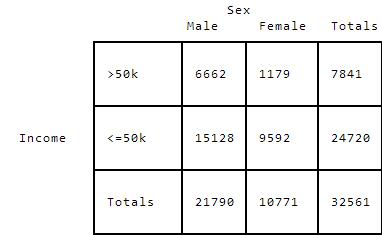sklearn.metrics.pairwise.euclidean_distances

python sklearn包中欧几里得距离计算的实现。 计算所应用的公式为dist(x, y) = sqrt(dot(x, x) - 2 * dot(x, y) + dot(y, y))，应用此公式有两点好处，第一：当X和Y为稀疏矩阵时，计算效率会...

2015/07/15 15:52
361
0
Overfitting

1: Introduction While exploring regression, we've briefly mentioned overfitting and the problems it can cause. In this mission, we'll explore how to identify overfitting and wha...

2016/09/28 05:09
17
0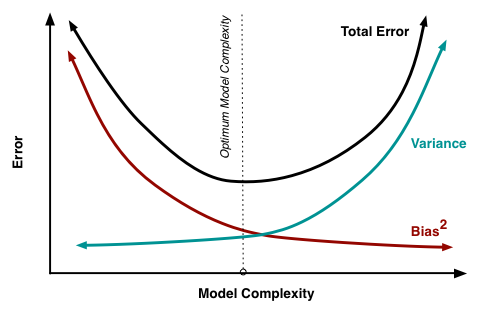Modeling Notes

1. Stats Standard deviation - how spread out the data is s = sqrt[ sum of power(Xi - Xmean, 2) / (n-1)], n-1 for sample, n for entire population Variance - square of SD Covarian...

2015/09/16 05:36
18
0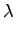WEKA summary含义记录

Correctly Classified Instaces，正确分类的instances。即预测准确度，最重要的评测指标。 Incorrectly Classified Instances，不正确。 Kappa statistic，评测观察员的差异（不懂）详细见下...

2012/09/07 11:18
204
0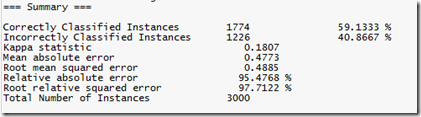Intermediate linear regression

1: Introduction To The Data The Leaning Tower of Pisa is one of the largest tourist attractions in Italy. For hundreds of years this tower slowly leaned to one side, eventually ...

2016/09/27 15:45
9
0Introduction to Machine Learning

1: Introduction To Machine Learning In data science, we're often trying to understand a process or system using observational data. Here are a few specific examples: How do the ...

2016/09/25 07:57
7
0Keras实践笔记1——线性回归

``` import matplotlib.pyplot as plt import numpy as np from keras import Sequential from keras.layers import Dense x = np.linspace(-10, 10, 300) y = 3 * x + np.random.random(x.s...

2018/06/09 23:07
10
0